## Introduction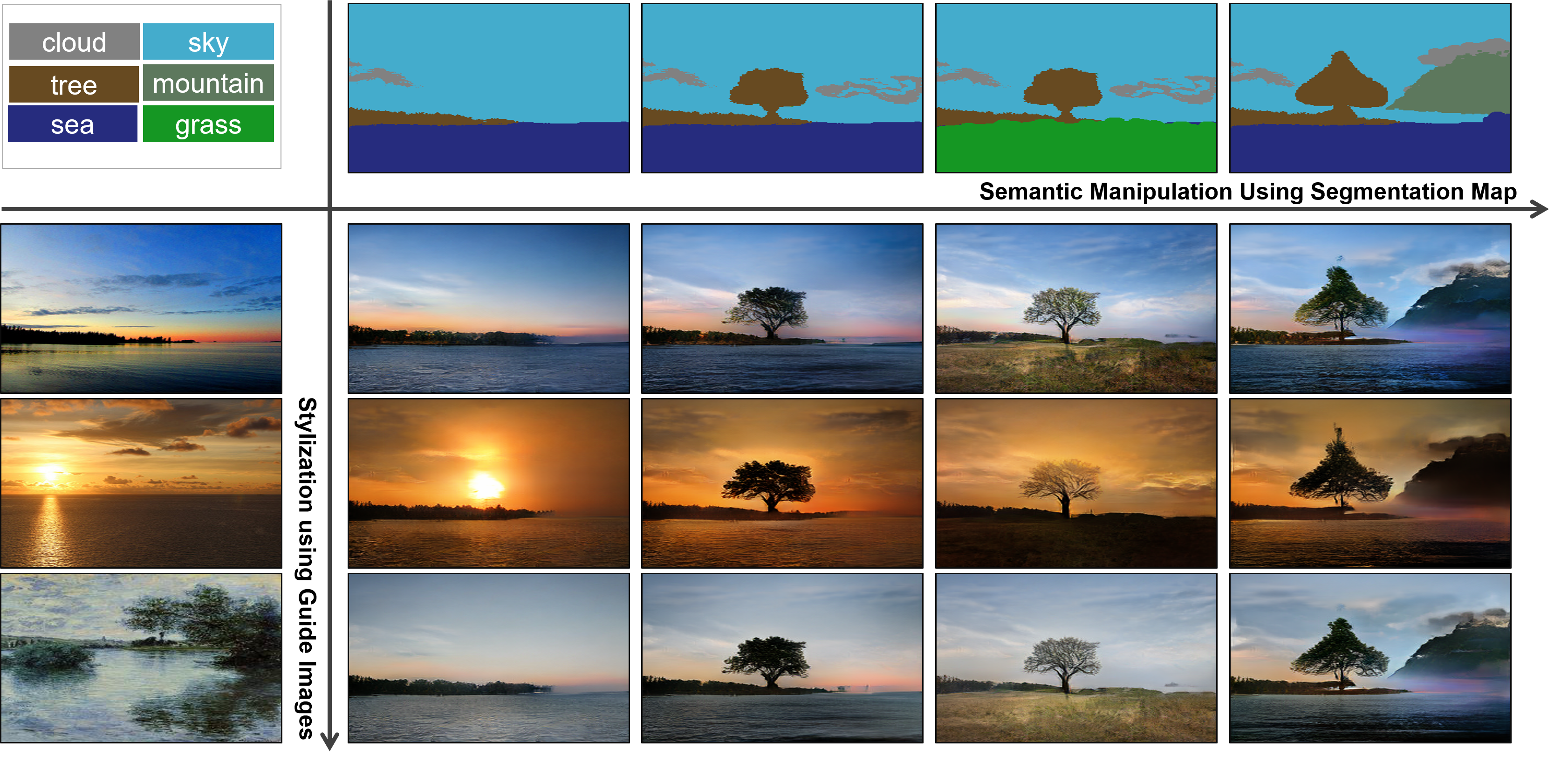Conventional convolution network architectures, using normalization layers, tend to “wash away” [sic] information in input semantic masks. Therefore, the authors mention that they are sub-optimal at best for semantic image synthesis.

The authors propose a new normalization layer called SPatially-Adaptative DEnormalization (SPADE) to overcome these limitations.

Whereas batch norm normalizes the output of an activation according to a unit Gaussian distribution, SPADE then denormalizes the output with learned scale $$\gamma$$ and bias $$\beta$$ in the form of a two-layer CNN.

The activation value after a SPADE layer at site $$i$$ is given by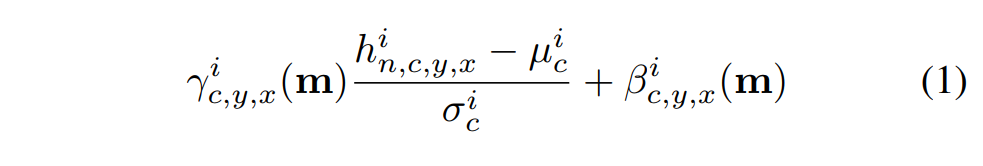where $$i$$ is the i-th pixel of the output; $$n, c, y, x$$ are the batch element, channel, y and x coordinates, $$m$$ the input segmentation mask, $$h$$ the output of the activation layer and $$\mu$$ and $$\sigma$$ are given by: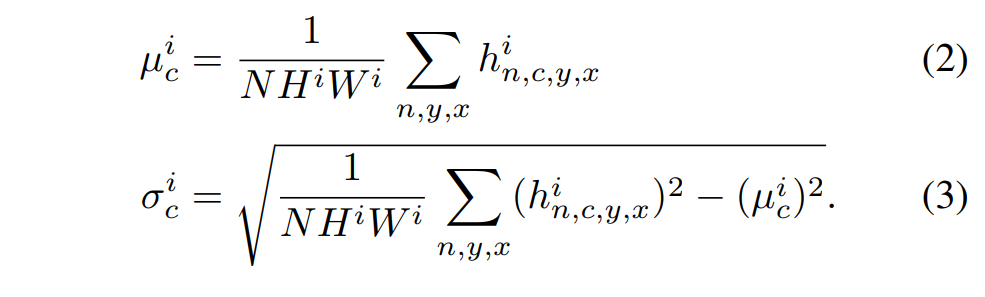Architecturally-speaking, it looks something like: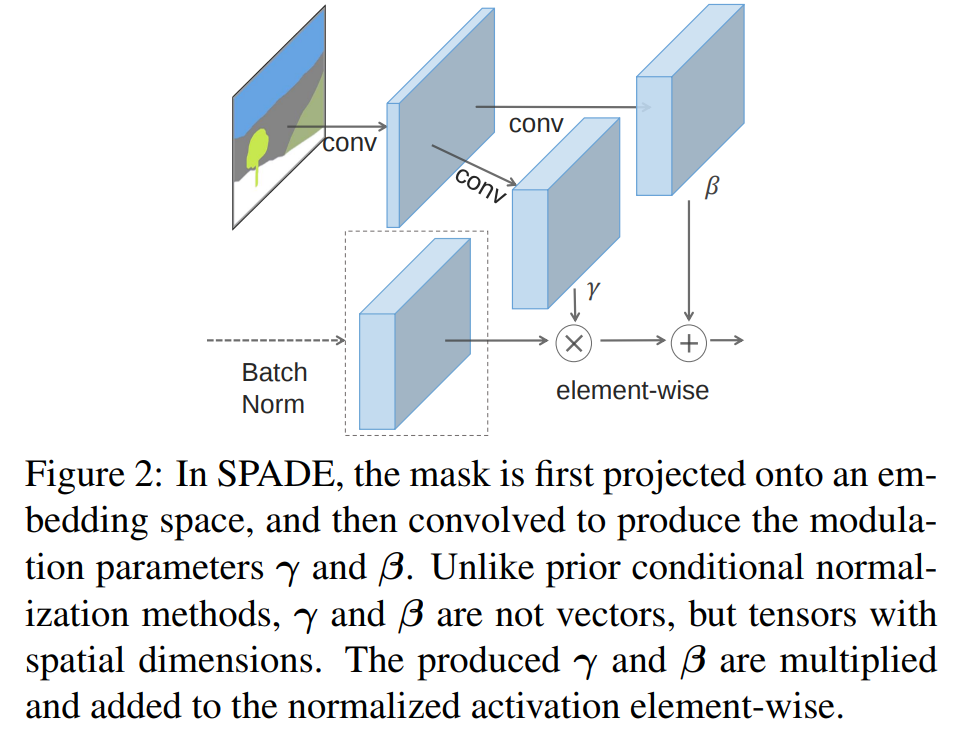The authors argue that it is a generalization of Conditional Batch Norm 1as SPADE would be the same if you replaced the segmentation mask with the image class label and made the modulation parameters spatially invariant ($$\gamma^i_{c,y_1,x_1} \equiv \gamma^i_{c,y_2,x_2}\equiv ...$$)

## Architecture

The architecture is quite simple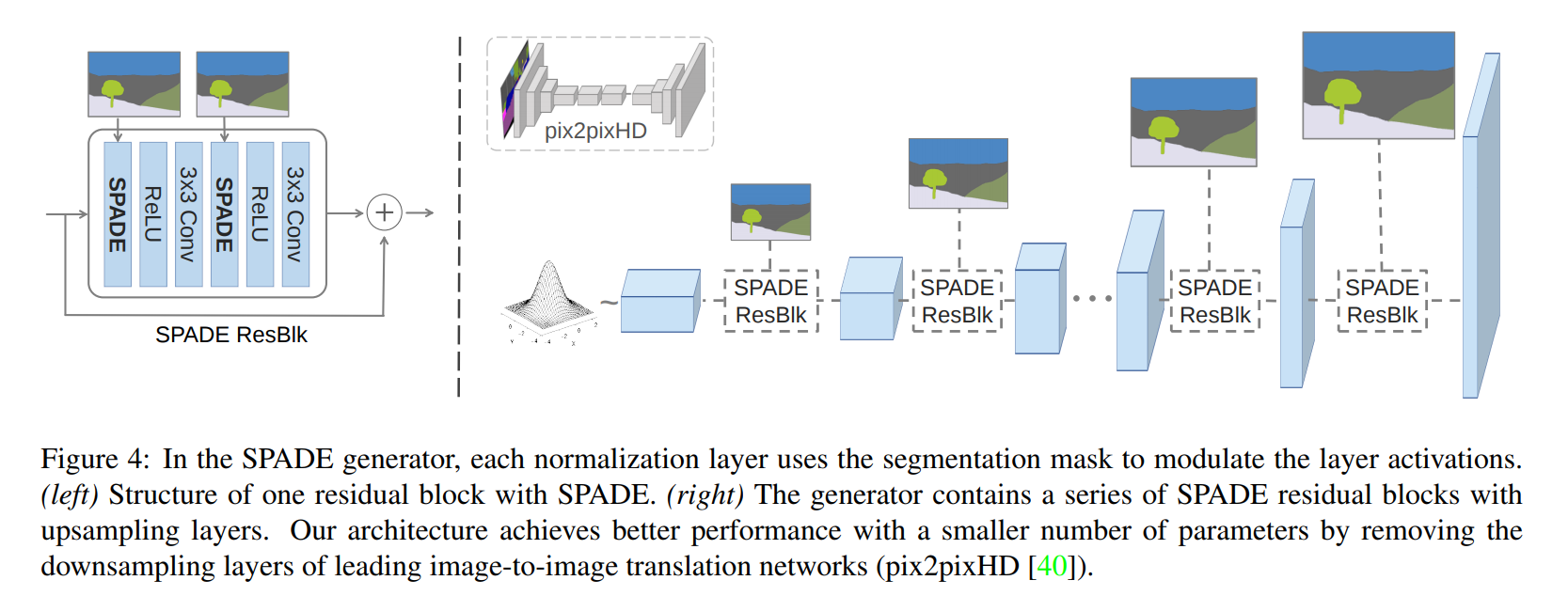The full structure of the networks is available in the paper.

## Results

They’re pretty wild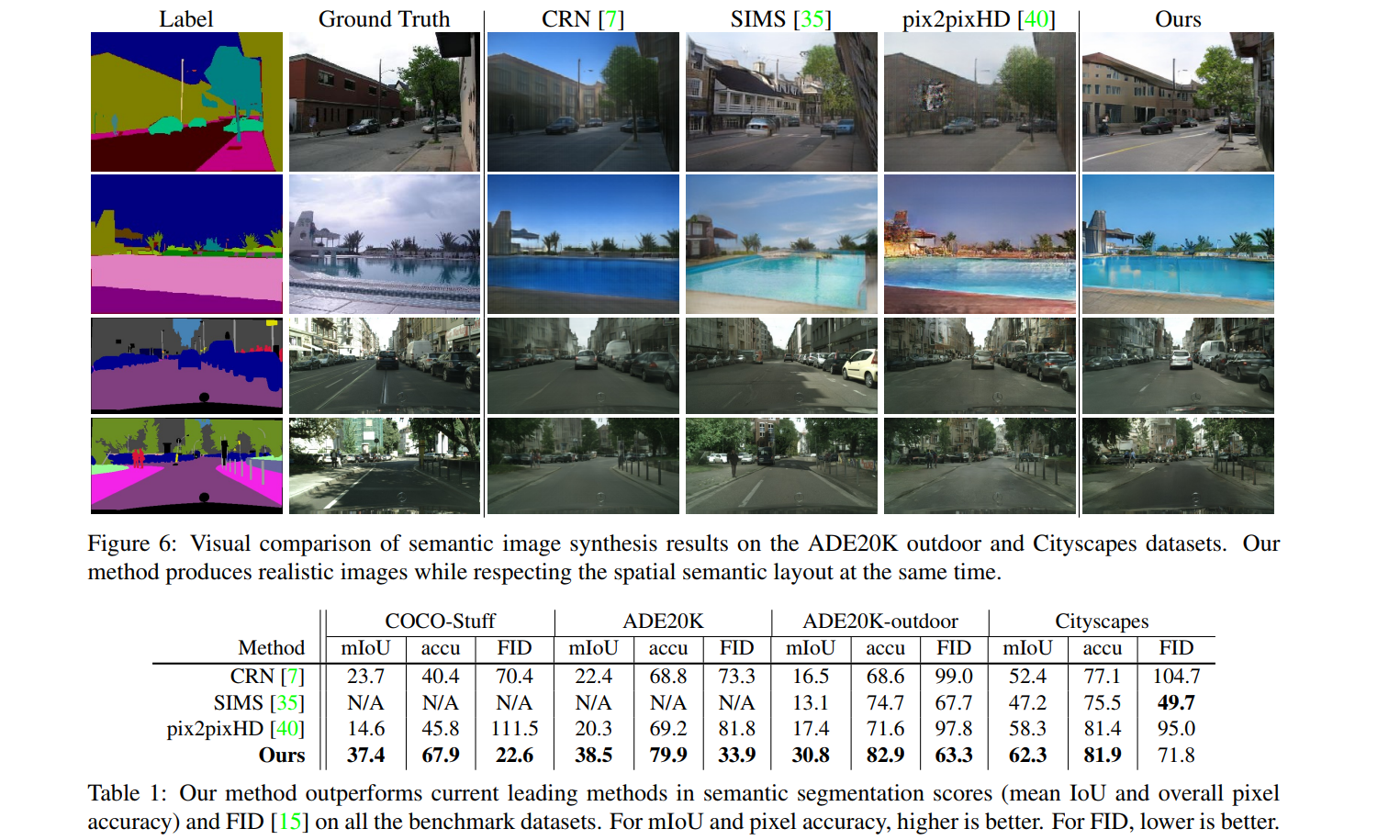A bunch of other metrics (such as qualitative evaluation results), as well as ablation and augmentation studies, are available in the paper.

1: Conditional Batch Norm: https://arxiv.org/pdf/1707.03017.pdf

Page (look at the video !)

Code